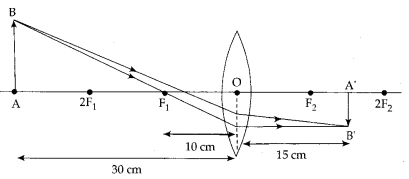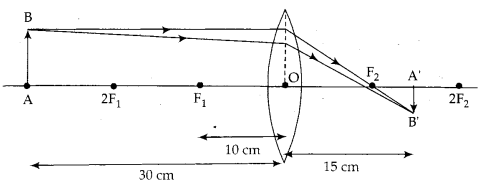# One half of a convex lens of focal length 10 cm

One half of a convex lens of focal length 10 cm is covered with a black paper. Can such a lens produce an image of a complete object placed at a distance of 30 cm from the lens? Draw a ray diagram to justify your answer.
A 4 cm tall object is placed perpendicular to the principal axis of a convex lens of focal length 20 cm. The distance of the object from the lens is 15 cm. Find the nature, position . and size of the image.

An image is formed from a number of rays from the object getting refracted by the lens. The complete image will be formed even if one half of the lens is covered. However, the brightness of the image will be less.

Case I: When the upper half of the lens is covered
In this case, rays of light coming from the object get refracted by the lower half of the lens. These rays meet at the other side of the lens to form the image of the given object as shown in the following figureCase II: When the lower half of the lens is covered.
In this case, rays of light coming from the object get refracted by the upper half of the lens. These rays meet at the other side of the lens to form the image of the given object as shown in the following figure:If the object is placed between the focus and the lens, then the image formed will be erect, virtual and enlarged. The image will be formed on the same side as object.
Size of the object, h0 = 4 cm
Focal length of the mirror, f = 20 cm
Object distance, u = -15 cm
Let the size of the image be hi and the image distance be v.
lf = 1 v - 1 u ⇒ 1 v = 1f + 1u ⇒ 1 v = 120 + 1 (-15) 1 v = -160v = -60 cm
Magnification of lens is given by m = v um = - 60 / 15m = 4.